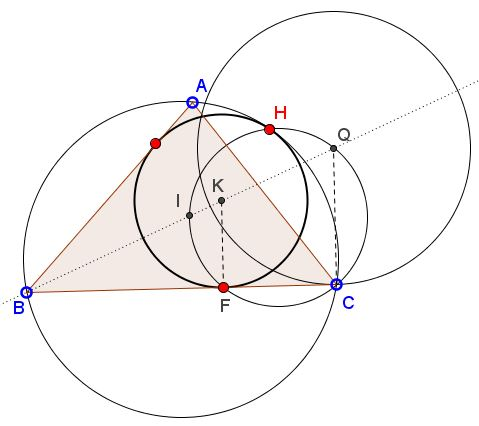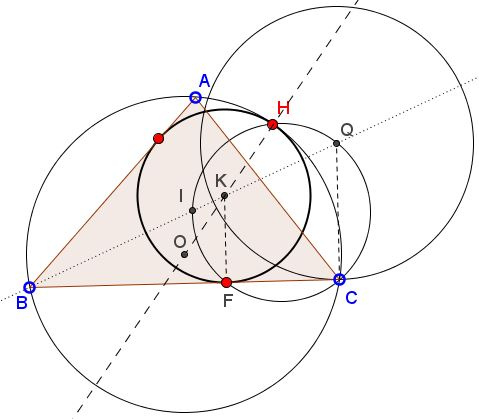# Construction and Properties of Mixtilinear Incircles 2

### Problem

Given $\Delta ABC,$ construct the mixtilinear incircle inscribed into angle $B.$### Solution

The construction proceeds in three steps:1. Construct circle $(Q)$ inscribed into angle $B$ and tangent to $BC$ at $C:$ draw the perpendicular to $BC$ at $C$ to its intersection at $Q$ with the angle bisector of $\angle B.$

2. Pass a circle through $C,$ $I$ the incenter of $\Delta ABC$, and $Q.$ Find $F$ - its second intersection with $BC.$

3. Errect the perpendicular at $F$ to $BC$. Its point of intersection $K$ with the bisector of $\angle B$ is the center of the sought mixtilinear circle.

### Proof

By the Power of a Point Theorem, $BF\cdot BC=BI\cdot BQ.$ Obviously, $\displaystyle\frac{BQ}{BI}=\frac{c}{p-b},$ where, as usual, $a,b,c$ are the side lengths of $\Delta ABC$ and $p$ its semiperimeter. We also know that $BI^{2}=\displaystyle\frac{(p-b)ac}{p}.$ From here,

$BF=BI^{2}\displaystyle\frac{1}{p-b}=\frac{ac}{p},$

exactly the expression for the point of tangency of the mixtilinear circle with $BC.$

### Extra

The second point of intersection of $(CIQ)$ with the circumcircle $(ABC)$ - $H$ in the diagram below, is the point of tangency of the circumcircle and the mixtilinear circle $(K).$ In other words, points $H,$ $K,$ and the circumcenter $O$ are collinear.### Acknowledgment

The construction has been posted by Dao Thanh Oai (Vietnam) at the CutTheKnotMath facebook page.

### Mixtilinear Incircles

• Mixtilinear Circles and Concurrence
• Sangakus with a Mixtilinear Circle
• Radius and Construction of a Mixtilinear Circle
• Sangaku with Three Mixtilinear Circles
• Construction and Properties of Mixtilinear Incircles
• Conic in Mixtilinear Incircles
• A Sangaku: Two Unrelated Circles
•